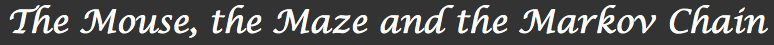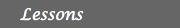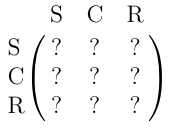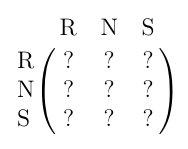1. Introduction2. The mouse and the maze 3. Processes, matrices and Markov chains 4. Will the mouse ever find the exit? How fast? 5. Other examples and applicationsOther examples and applications

Following are examples and applications that have been collected from the internet, clipped, adjusted and/or rewritten to suit our needs and notations. Nothing is original in here, but the author believes that the situations described were interesting enough to be included here. All of these exercises are probably better carried on with the aid of a computer or a graphing calculator.

## 1. Amusement Park

An amusement park has five adult attractions: Roller coaster, Flume, Wheel, Smasher and Crunch. Two of these, the exciting Roller coaster and Flume, are usually the last attractions visited before people leave the park. There is a path between the Roller coaster and Flume, Roller coaster and Wheel, Roller coaster and Smasher, Flume and Wheel, Flume and Crunch, Smasher and Wheel, and Wheel and Crunch.

Question
Assuming that people are equally likely to take any path leaving an attraction and that 25% of the people who ride the Roller coaster or the Flume then leave the park, draw the graph that shows the five attractions and the exit and shows the probability that someone at one vertex goes to any other vertex. Use E for exit, R for roller coaster and so on. Assume that someone who has exited the park does not return.
Question
From this graph, write the transition probability matrix P for going from one attraction to another. The entries in column E would be 0 everywhere except at row E to indicate that someone who exits the park does not return (”stays out”).
Question
Use this matrix to determine the probability that a person who rides the Roller Coaster rides it once again after four changes of rides. To do this, you need to create an initial state vector q with zeroes everywhere expect at row R indicating you started on the Roller Coaster. Multiply P and q to determine the probability distribution for the next ride. Keep multiplying by P to get subsequent observations.
Question
What is the probability that someone who is now riding the Roller Coaster rides the Wheel after changing rides four times?
Question
Find the expected number of times that someone who is now riding the Roller Coaster rides the Wheel before leaving the park.
Question
Find the expected number of rides that a person who is now riding the roller coaster rides on any ride whatsoever before leaving the park.

## 2. Genetics

A given plant species has red, pink, or white flowers according to the genotypes RR, RW, and WW respectively. If each genotype is crossed with a pink flowering plant (genotype RW), the transition matrix is as follows:These numbers are determined as follows:
1. RR x RW yields RR, RW, RR, and RW.
2. RW x RW yields RR, RW, WR, WW.
3. WW x RW yields WR, WW, WR, and WW.
Assume the plants of each generation are crossed only with pink plants to produce the next generation.

Question
This is the transition matrix is P. Now create a state vector q indicating an initial distribution of genotypes of 70% Red, 10% Pink, and 20% White.
Question
Determine the distribution of phenotypes for the next generation starting from q. Multiply the answer with P and keep multiplying. Eventually the matrix product will stabilize. What does this show? What will be the eventual distribution of genotypes after many generations?
Question
Suppose the initial distribution is different. Modify the values in vector q to indicate a different distribution (remember the three numbers must add up to 1) and repeat the multiplications as in the previous question. Explain your results.
Question
Suppose we decide to cross plants of each generation with only white plants. Create a transition matrix for this situation. (To figure the probabilities, recall that RR x WW yields RW all the time. Calculate the other probabilities.) Use the same initial distribution of genotypes and determine the eventual distribution after many generations.

## 3. Predicting the Weather

Suppose a weather forecaster has collected data to predict whether tomorrow will be sunny (S), cloudy (C), or rainy (R), given today’s weather conditions. Here is a summary of his analysis:
• If today is sunny, then tomorrow’s probabilities are 70% for S, 20% for C, and 10% for R.
• If today is cloudy, then the probabilities for tomorrow are 30% for S, 60% for C, and 10% for R.
• If today is cloudy, then the probabilities for tomorrow are 25% for S, 20% for C, and 55% for R.
Question
With this information form a Markov chain describing the weather changes. Take as states the kinds of weather S, C, and R. From the above information determine the transition probabilities:Question
Start with today’s weather and determine the probabilities for tomorrow’s weather. Multiply by the transition matrix P again to determine the weather for 2 days from now. Repeat this so that you have a set of probabilities for the next 5 days.
Question
Keep multiplying by P. Eventually the matrix product will stabilize. What does this show?
Question
Question Change today’s weather to indicate a rainy day. Repeat the multiplications as before to formulate a 5-day forecast.
Question
Now forget about state vectors and just take P and raise it to some power, such as 20. What do you see? What does that tell you about the significance the state vector here? For what value of n does Pn stabilize? Once it stabilizes it no longer forecasts the weather, but rather tells you about the climate.
Question
Question Create a matrix that would be appropriate for Ithaca, NY. You may want to include snow as well as rain. Decide on today’s weather and illustrate a 5-day forecast.

## 4. Predicting the Weather: The Land of Oz

According to Kemeny, Snell, and Thompson, the Land of Oz is blessed by many things, but not by good weather. They never have two nice days in a row. If they have a nice day, they are just as likely to have snow as rain the next day. If they have snow or rain, they have an even chance of having the same the next day. If there is change from snow or rain, only half of the time is this a change to a nice day.

Question
With this information form a Markov chain describing the weather changes. Take as states the kinds of weather R, N, and S. From the above information determine the transition probabilities:Question
Study the climate of the Land of Oz. If you don’t understand this question, make sure you read the previous example.